Anúncio

# Data types.pdf

4 de Sep de 2022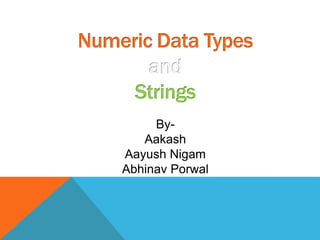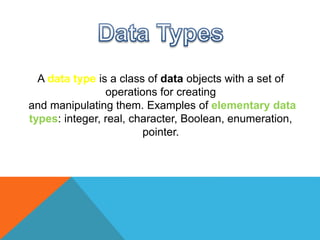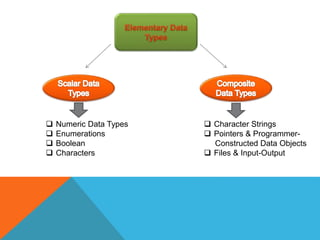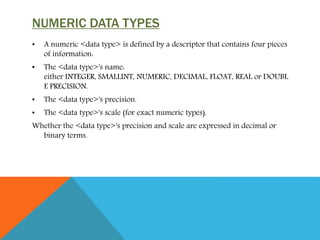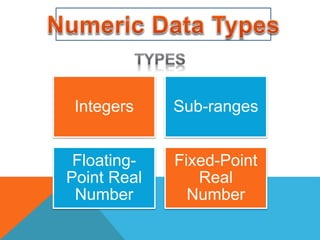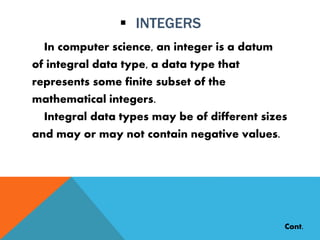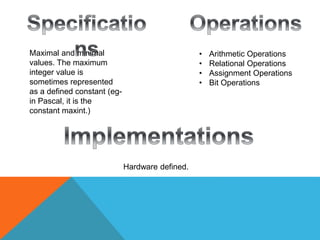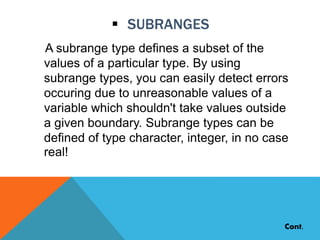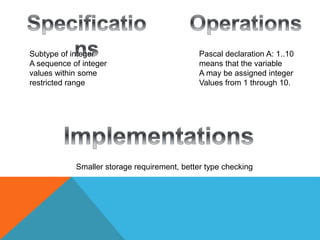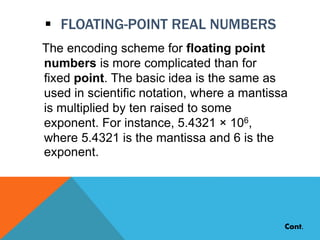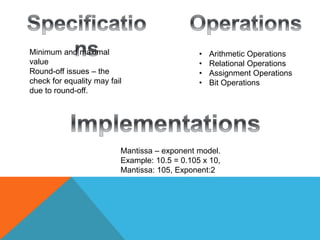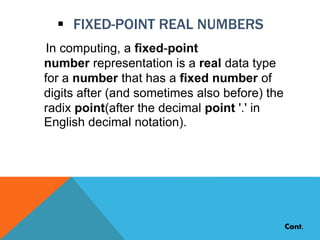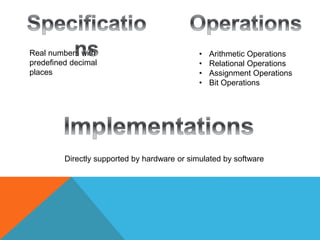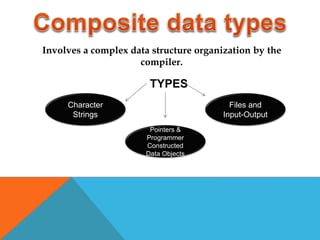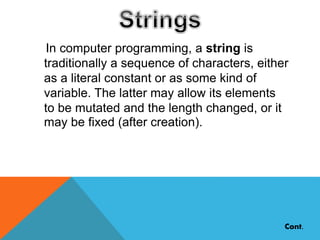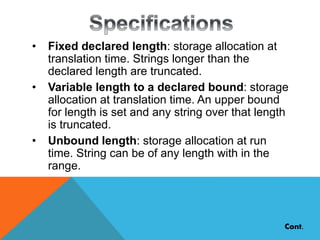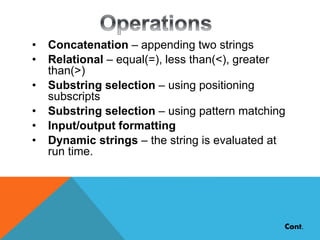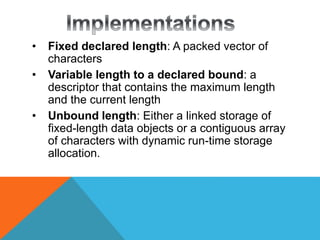1 de 19
Anúncio

### Data types.pdf

1. By- Aakash Aayush Nigam Abhinav Porwal
2. A data type is a class of data objects with a set of operations for creating and manipulating them. Examples of elementary data types: integer, real, character, Boolean, enumeration, pointer.
3.  Numeric Data Types  Enumerations  Boolean  Characters  Character Strings  Pointers & Programmer- Constructed Data Objects  Files & Input-Output
4. NUMERIC DATA TYPES • A numeric <data type> is defined by a descriptor that contains four pieces of information: • The <data type>'s name: either INTEGER, SMALLINT, NUMERIC, DECIMAL, FLOAT, REAL or DOUBL E PRECISION. • The <data type>'s precision. • The <data type>'s scale (for exact numeric types). Whether the <data type>'s precision and scale are expressed in decimal or binary terms.
5. Integers Sub-ranges Floating- Point Real Number Fixed-Point Real Number
6.  INTEGERS In computer science, an integer is a datum of integral data type, a data type that represents some finite subset of the mathematical integers. Integral data types may be of different sizes and may or may not contain negative values. Cont.
7. Maximal and minimal values. The maximum integer value is sometimes represented as a defined constant (eg- in Pascal, it is the constant maxint.) • Arithmetic Operations • Relational Operations • Assignment Operations • Bit Operations Hardware defined.
8.  SUBRANGES A subrange type defines a subset of the values of a particular type. By using subrange types, you can easily detect errors occuring due to unreasonable values of a variable which shouldn't take values outside a given boundary. Subrange types can be defined of type character, integer, in no case real! Cont.
9. Subtype of integer A sequence of integer values within some restricted range Pascal declaration A: 1..10 means that the variable A may be assigned integer Values from 1 through 10. Smaller storage requirement, better type checking
10.  FLOATING-POINT REAL NUMBERS The encoding scheme for floating point numbers is more complicated than for fixed point. The basic idea is the same as used in scientific notation, where a mantissa is multiplied by ten raised to some exponent. For instance, 5.4321 × 106, where 5.4321 is the mantissa and 6 is the exponent. Cont.
11. Minimum and maximal value Round-off issues – the check for equality may fail due to round-off. • Arithmetic Operations • Relational Operations • Assignment Operations • Bit Operations Mantissa – exponent model. Example: 10.5 = 0.105 x 10, Mantissa: 105, Exponent:2
12.  FIXED-POINT REAL NUMBERS In computing, a fixed-point number representation is a real data type for a number that has a fixed number of digits after (and sometimes also before) the radix point(after the decimal point '.' in English decimal notation). Cont.
13. Real numbers with predefined decimal places • Arithmetic Operations • Relational Operations • Assignment Operations • Bit Operations Directly supported by hardware or simulated by software
14. Involves a complex data structure organization by the compiler. Character Strings Pointers & Programmer Constructed Data Objects Files and Input-Output TYPES
15. In computer programming, a string is traditionally a sequence of characters, either as a literal constant or as some kind of variable. The latter may allow its elements to be mutated and the length changed, or it may be fixed (after creation). Cont.
16. Cont. • Fixed declared length: storage allocation at translation time. Strings longer than the declared length are truncated. • Variable length to a declared bound: storage allocation at translation time. An upper bound for length is set and any string over that length is truncated. • Unbound length: storage allocation at run time. String can be of any length with in the range.
17. Cont. • Concatenation – appending two strings • Relational – equal(=), less than(<), greater than(>) • Substring selection – using positioning subscripts • Substring selection – using pattern matching • Input/output formatting • Dynamic strings – the string is evaluated at run time.
18. • Fixed declared length: A packed vector of characters • Variable length to a declared bound: a descriptor that contains the maximum length and the current length • Unbound length: Either a linked storage of fixed-length data objects or a contiguous array of characters with dynamic run-time storage allocation.
Anúncio